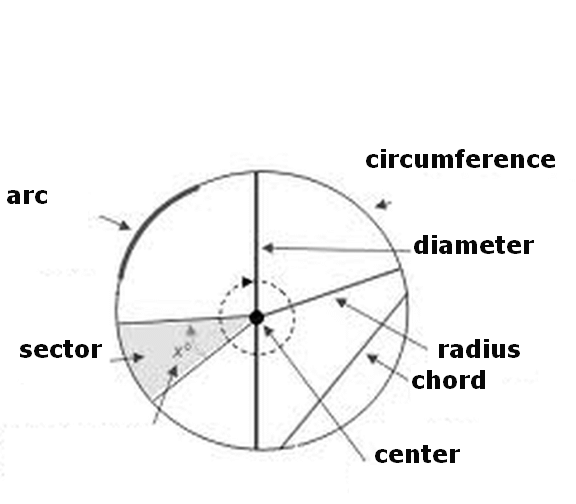### Circle

The circle is closed curve figure where all the points on the curve has the same distance from the center point.
Circle components:
Radius: straight line segment joining from center to any part of the curve;
Chords: any straight line segments joining two points of de curve;
Diameter is a chord that passes through the center of the circle;
Arc: a curve line segment;
Apothem: the height of the triangle formed, with the connections of the ends of the chord and the center curve;
Circumference: the length curve.
Sagitta: a straight line connecting the midpoints of the curve to the center of its base. It is also the height of an arc. Consequently the radius is also the height of a semicircle.
The division of the circumference by the diameter is always equal to the constant Π (Pi), that is 3.14 ...
Enter data using the point as a decimal separator. Ex 1,250.37 enter: 1250.37; the results will be shown after a click on Calculate.Circle Decimal places: Radius: Chord AB: Diameter: Arc:    Sagitta: Circumference: Circle area: Sector area: Segment area: Triangle area: Apothem: Sagitta: Arc: Central angle: Angle A: Pi: Note:

Note:The accuracy of the calculator and its applicability to particular cases is not guaranteed.